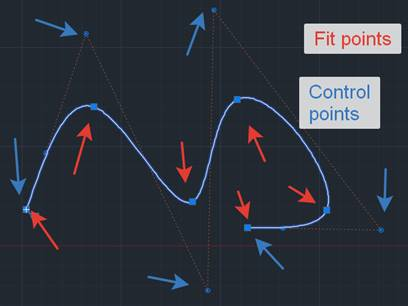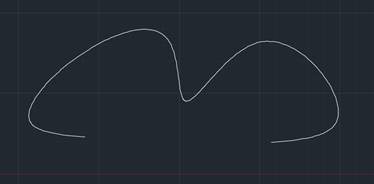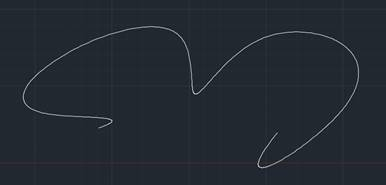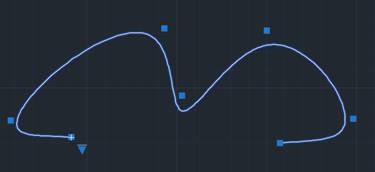# Creating a NURBS Curve

May 17, 2018

With Teigha you can create Non-Uniform Rational Basis Spline (NURBS) curves using the Teigha Ge library and its classes OdGeNurbCurve2d and OdGeNurbCurve3d.

Let’s consider a 3D version of a curve (2D curves have all the same behavior). A NURBS curve is defined by its order, a set of weighted control points, and a knot vector. This data can be set to a curve using a constructor:

``````OdGeNurbCurve3d(
int degree,
const OdGeKnotVector& knots,
const OdGePoint3dArray& controlPoints,
const OdGeDoubleArray& weights,
bool isPeriodic = false);
``````

But this is “core” mathematical data describing NURBS; it is not intuitive to use it to create a shape that you might need. The other option is to create a NURBS using fit data. This is the constructor that sets the fit data:

``````OdGeNurbCurve3d(
const OdGePoint3dArray& fitPoints,
const OdGeVector3d& startTangent,
const OdGeVector3d& endTangent,
bool startTangentDefined = true,
bool endTangentDefined = true,
const OdGeTol& fitTol = OdGeContext::gTol);
``````

Here we use fitPoints which are interpolation points that the curve will go through. This means that fit points are on the curve. The following picture shows the difference between fit points and control points:Start and end tangents are vectors that define tangents at the ends of the curve. You cannot define them by setting corresponding flags to ‘false’. The fit tolerance is a distance that the curve may deviate from the fit points. By default it is zero and the curve passes exactly through all the fit points. The following pictures show the influence of these parameters:End tangents are not definedEnd tangents are definedFit tolerance is defined, and the curve passes ‘near’ the fit points

Also you can create a NURBS with fit points that define a tangent in each fit point. Use the following constructor:

``````OdGeNurbCurve3d(
const OdGePoint3dArray& fitPoints,
const OdGeVector3dArray& fitTangents,
const OdGeTol& fitTolerance = OdGeContext::gTol,
bool isPeriodic = false);
``````

And you can create fit data to use even if the curve was created initially using control points and knots; use the function buildFitData.

For database purposes, you can use the OdDbSpline class to represent a 3D NURBS curve as well. You can create an OdGeNurbCurve3d curve of the shape you need and set it to the OdDbSpline class using the setFromOdGeCurve method.